• matlab给图像添加噪声
千次阅读
2021-04-18 07:02:16

如何给图像添加高斯白噪声

噪声中有两种性质的噪声，加性噪声和乘性噪声。白噪声(白杂讯)，是一种功率频谱密度为常数的随机信号或随机过程，是功率谱密度在整个频域内均匀分布的噪声。此信号在各个频段上的功率是一样的，由于白光是由各种频率(颜色)的单色光混合而成，因而此信号的这种具有平坦功率谱的性质被称作是“白色的”，此信号也因此被称作白噪声。相对的，其他不具有这一性质的噪声信号(功率谱密度不均匀分布)被称为有色噪声。

一个噪声过程所具有的频谱宽度远远大于它所作用系统的带宽。理想的白噪声具有无限带宽，因而其能量是无限大，这在现实世界是不可能存在的。实际上，我们常常将有限带宽的平整讯号视为白噪音，因为这让我们在数学分析上更加方便。然而，白噪声在数学处理上比较方便，因此它是系统分析的有力工具。一般，只要一个噪声过程所具有的频谱宽度远远大于它所作用系统的带宽，并且在该带宽中其频谱密度基本上可以作为常数来考虑，就可以把它作为白噪声来处理。例如，热噪声和散弹噪声在很宽的频率范围内具有均匀的功率谱密度，通常可以认为它们是白噪声。

加性噪声一般指热噪声、散弹噪声等，它们与信号的关系是相加，不管有没有信号，噪声都存在。而乘性噪声一般由信道不理想引起，它们与信号的关系是相乘，信号在它在，信号不在他也就不在。一般通信中把加性随机性看成是系统的背景噪声；而乘性随机性看成系统的时变性(如衰落或者多普勒)或者非线性所造成的。

MATLAB中产生高斯白噪声非常方便，可以直接应用两个函数，一个是WGN，另一个是AWGN。WGN用于产生高斯白噪声，AWGN则用于在某一信号中加入高斯白噪声。

1. WGN：产生高斯白噪声

y = wgn(m,n,p) 产生一个m行n列的高斯白噪声的矩阵，p以dBW为单位指定输出噪声的强度。

y = wgn(m,n,p,imp) 以欧姆(Ohm)为单位指定负载阻抗。

y = wgn(m,n,p,imp,state) 重置RANDN的状态。

在数值变量后还可附加一些标志性参数：

y = wgn(…,POWERTYPE) 指定p的单位。POWERTYPE可以是'dBW',

'dBm'或'linear'。线性强度(linear power)以瓦特(Watt)为单位。

y = wgn(…,OUTPUTTYPE) 指定输出类型。OUTPUTTYPE可以是'real'或'complex'。

2. AWGN：在某一信号中加入高斯白噪声

y = awgn(x,SNR)

在信号x中加入高斯白噪声。信噪比SNR以dB为单位。x的强度假定为0dBW。如果x是复数，就加入复噪声。

y = awgn(x,SNR,SIGPOWER)

如果SIGPOWER是数值，则其代表以dBW为单位的信号强度；如果SIGPOWER为'measured'，则函数将在加入噪声之前测定信号强度。

y = awgn(x,SNR,SIGPOWER,STATE) 重置RANDN的状态。

y = awgn(…,POWERTYPE)

指定SNR和SIGPOWER的单位。POWERTYPE可以是'dB'或'linear'。如果POWERTYPE是'dB'，那么SNR以dB为单位，而SIGPOWER以dBW为单位。如果POWERTYPE是'linear'，那么SNR作为比值来度量，而SIGPOWER以瓦特为单位。

注释

1. 分贝(decibel,

dB)：分贝(dB)是表示相对功率或幅度电平的标准单位，换句话说，就是我们用来表示两个能量之间的差别的一种表示单位，它不是一个绝对单位。例如，电子系统中将电压、电流、功率等物理量的强弱通称为电平，电平的单位通常就以分贝表示，即事先取一个电压或电流作为参考值(0dB)，用待表示的量与参考值之比取对数，再乘以20作为电平的分贝数(功率的电平值改乘10)。

2. 分贝瓦(dBW, dB Watt)：指以1W的输出功率为基准时，用分贝来测量的功率放大器的功率值。

3. dBm (dB-milliWatt)：即与1milliWatt(毫瓦)作比较得出的数字。

0 dBm = 1 mW

10 dBm = 10 mW

20 dBm = 100 mW

也可直接用randn函数产生高斯分布序列，例如：

y=randn(1,2500);

y=y/std(y);

y=y-mean(y);

a=0.0128;

b=sqrt(0.9596);

y=a+b*y;

就得到了 N ( 0.0128, 0.9596 ) 的高斯分布序列

产生指定方差和均值的随机数

设某个随机变量x均值为mu，方差为var^2，若要产生同样分布的随机变量y，但使新的随

机变量参数改变，均值为mu_1，方差为var_1^2，可以用如下公式进行变换：

y=var_1/var*(x-mu)+mu_1，其中x为随机变量，其余为常数(原分布参数)。

具体到正态分布，若要产生均值为u，方差为o^2的M*N的随机数矩阵，可以用

y=o*randn(M,N)+u得到。

对于均匀分布，若要产生[a,b]区间的均匀分布的M*N的随机数矩阵，则可以用

y=rand(M,N)*(b-a)+a得到。

%=========================================%

上述资料基本上完整地描述了原始问题，不过有几点内容附带说明一下：

1. 上面资料最后部分隐含了一个出自zhyuer 版友的结论：

%=========================================%

1)  rand产生的是[0，1]上的均匀分布的随机序列

2)  randn产生均值为0，方差为1的高斯随机序列，也就是白噪声序列；

%=========================================%

也就是说，可以直接使用上面两个函数对原始信号添加噪声(例如y=x+rand(length(x),1)或者y=x+randn(length(x),1))

2.

事实上，无论是wgn还是awgn函数，实质都是由randn函数产生的噪声。即，wgn函数中调用了randn函数，而awgn函数中调用了wgn函数。下面就我熟悉的“向已知信号添加某个信噪比(SNR)的高斯白噪声”来说明一下，不过如果大家阅读过awgn的实现代码就不用看下去了，呵呵。从上述可知，这个任务可以使用awgn函数实现，具体命令是：awgn(x,snr,’measured’,'linear’)，命令的作用是对原信号f(x)添加信噪比(比值)为SNR的噪声，在添加之前先估计信号f的强度。这里涉及三个问题：在awgn这个函数中，SNR是如何计算的？什么是信号的强度？awgn函数具体是如何添加噪声的？事实上，前两个问题是相关的，因为根据定义，SNR就是信号的强度除以噪声的强度，所以，首先来讲讲信号的强度。其实信号的强度指的就是信号的能量，在连续的情形就是对f(x)平方后求积分，而在离散的情形自然是求和代替积分了。在matlab中也是这样实现的，只不过多了一个规范化步骤罢了：

sigPower = sum(abs(sig(:)).^2)/length(sig(:))

这就是信号的强度。至此，SNR的具体实现也不用多说了(注：由于采用的是比值而非db，所以与下面“计算信噪比”所使用的方式不同，即没有求对数步骤)。

最后说说awgn函数具体是如何添加噪声的。事实上也很简单，在求出f的强度后，结合指定的信噪比，就可以求出需要添加的噪声的强度noisePower=sigPower/SNR。由于使用的是高斯白噪声即randn函数，而randn的结果是一个强度为1的随机序列(自己试试sum(randn(1000,1).^2)/1000就知道了，注意信号的长度不能太小)。于是，所要添加的噪声信号显然就是：sqrt(noisePower)*randn(n,1)，其中n为信号长度。

3. 上面所说的都是具有分布特性(相关的)随机序列，如果需要添加不相关的随机序列，则可以使用jimin版友的方法：

%=========================================%

for i=1:100

x(i)=randn(1);

end

%=========================================%

即先产生噪声信号，后再与原信号叠加。

最后是另外的一些常见问题，整理如下：

1. Matlab中如何产生值为0，1的随机序列？【转bainhome版友】：round(rand(5))

2. Matlab中如何计算信噪比？下面的代码转自Happy教授：

%=========================================%

function snr=SNR(I,In)

% 计算信号噪声比函数

% by Qulei

% I :original signal

% In:noisy signal(ie. Original signal + noise signal)

% snr=10*log10(sigma2(I2)/sigma2(I2-I1))

[row,col,nchannel]=size(I);

snr=0;

if nchannel==1%gray image

Ps=sum(sum((I-mean(mean(I))).^2));%signal power

Pn=sum(sum((I-In).^2));%noise power

snr=10*log10(Ps/Pn);

elseif nchannel==3%color image

for i=1:3

Ps=sum(sum((I(:,:,i)-mean(mean(I(:,:,i)))).^2));%signal power

Pn=sum(sum((I(:,:,i)-In(:,:,i)).^2));%noise power

snr=snr+10*log10(Ps/Pn);

end

snr=snr/3;

end

%=========================================%

3. 随机产生1-n的索引排列：randperm函数

4. 随机产生1-60的正整数一个(三种方法)：

A=randperm(60);

b=A(1)

A=round(100*rand(1,10))

b=A(1)

b =

unidrnd(60,1,1)

更多相关内容
• matlab添加多种噪声的实现方法

# 个人记录

# 个人记录

m=256; n=256;%图像大小

a=0.04;

k=-1/a;

I=k*log(1-rand(m, n));%指数分布噪声

figure;

subplot(121);  imshow(uint8(I)),title('指数分布噪声');

subplot(122);  imhist(uint8(I)),title('指数分布噪声');
% 【下面为matlab自带函数exprnd，生成指数随机数的公式】

% % Generate uniform random values, and apply the exponential inverse CDF.

% r = -mu .* log(rand(sizeOut, 'like', mu)); % == expinv(u, mu)

m=256; n=256;%图像大小

a=50;

b=180;

I=a+(b-a)*rand(m,n);%均匀分布噪声

figure;

subplot(121);  imshow(uint8(I)),title('均匀分布噪声');

subplot(122);  imhist(uint8(I)),title('均匀分布噪声');
%通过均值和方差产生高斯噪声

I=uint8(100*ones(256, 256));%均值为100

J=imnoise(I, 'gaussian', 0, 0.01);%高斯噪声 方差0.01

K=imnoise(I, 'gaussian', 0, 0.03);

figure;

subplot(121);  imshow(J),title('高斯噪声 方差0.01');

subplot(122);  imhist(J),title('高斯噪声 方差0.01');
figure;

subplot(121);  imshow(K),title('高斯噪声 方差0.03');

subplot(122);  imhist(K),title('高斯噪声 方差0.03');
%通过位置信息产生高斯噪声

I=im2double(I);

V=zeros(size(I));%建立矩阵V

for i=1:size(V, 1)

V(i,:)=0.02*i/size(V,1);

end

J=imnoise(I, 'localvar', V);%添加高斯噪声 V为与I大小相同的数组

figure;

subplot(121);  imshow(I),title('原图像');

subplot(122);  imshow(J),title('添加高斯噪声后图像');
%根据亮度值产生高斯噪声

I=im2double(I);

h=0:0.1:1;

v=0.01:-0.001:0;

J=imnoise(I, 'localvar', h, v);%h为在[0,1]之间的向量 表示图像的亮度值 v为一个长度和h相同，表示与h中亮度对应的高斯噪声的方差

figure;

subplot(121);  imshow(I),title('原图像');

subplot(122);  imshow(J),title('添加高斯噪声后的图像');
I=im2double(I);

J=imnoise(I, 'salt & pepper', 0.01);%0.01为噪声密度

K=imnoise(I, 'salt & pepper', 0.03);

figure;

subplot(121);  imshow(J),title('椒盐噪声，噪声密度0.01');

subplot(122);  imshow(K),title('椒盐噪声，噪声密度0.03');
I=im2double(I);

R=rand(size(I));

J=I;

J(R<=0.02)=0;%添加椒噪声

K=I;

K(R<=0.03)=1;%添加盐噪声

figure;

subplot(121);  imshow(J),title('含椒噪声');

subplot(122);  imshow(K),title('含盐噪声');
% 添加Speckle noisemultiplicative noise

J=imnoise(I, 'speckle');%方差默认值

K=imnoise(I, 'speckle', 0.2);%方差0.2

figure;

subplot(121);  imshow(J),title('添加斑点噪声，方差0.05');

subplot(122);  imshow(K),title('添加斑点噪声，方差0.2');
J=imnoise(I, 'poisson');

figure;

subplot(121);  imshow(I),title('原始图像');

subplot(122);  imshow(J),title('添加泊松噪声');
展开全文matlab python
• 【仿真研究】给图片添加了正弦噪声，利用MATLAB源代码消除噪声.rar
• 文章目录噪声模型1 噪声种类1 .1 高斯噪声1.2 瑞利噪声1.3 伽玛噪声1.4 指数噪声1.5 均匀分布噪声1.6 脉冲噪声（椒盐噪声噪声模型 图像噪声主要来源于图像的获取和传输过程。 （1）图像传感器的工作情况受各种...

# 关注微信公众号【二进制人工智能】，回复【noise】获取所有代码

图像噪声主要来源于图像的获取和传输过程。

（1）图像传感器的工作情况受各种因素的影响，如图像获取中的环境条件和传感元器件自身的质量。例如，当使用CCD摄像机获取图像时，光照强度和传感器的温度是生成图像中产生大量噪声的主要因素。

（2）图像在传输过程中主要由于所用传输信道被干扰而受到噪声污染。比如，通过无线网络传输的图像可能会因为光或其他大气因素的干扰被污染。

## 1 噪声种类

### 1 .1 高斯噪声

高斯噪声是理论研究中最常见的噪声。一般而言，对一个抗噪系统而言高斯噪声是最恶劣的噪声，设计系统时只要能够抵抗高斯噪声，那么系统性能就有保证。

高斯噪声也是现实生活中极为常见的。根据中心极限定理，在自然界中，一些现象受到许多相互独立的随机因素的影响，如果每个因素所产生的影响都很微小，那么总的影响可以看作是服从正态分布的。

高斯随机变量z的概率密度函数由下式给出：
p ( z ) = 1 2 π σ e − ( z − μ ) 2 / 2 σ 2 p(z)=\frac{1}{\sqrt{2 \pi} \sigma} e^{-(z-\mu)^{2} / 2 \sigma^{2}}

其中， z z 表示灰度值， μ μ 表示 z z 的平均值或期望值， σ σ 表示 z z 的标准差。标准差的平方 σ 2 σ^2 称为 z z 的方差。

### 1.2 瑞利噪声

当一个随机二维向量的两个分量呈独立的、有着相同的方差的正态分布时，这个向量的模呈瑞利分布。服从这种分布的噪声即瑞利噪声，其概率密度函数由下式给出

p ( z ) = { 2 b ( z − a ) e − ( z − a ) 2 / b z ⩾ a 0 z < a p(z)=\left\{\begin{array}{ll}\frac{2}{b}(z-a) \mathrm{e}^{-(z-a)^{2 / b}} & z \geqslant a \\0 & z<a\end{array}\right.

概率密度的均值为：
μ = a + p b / 4 \mu=a+\sqrt{p b / 4}

方差为：
S 2 = b ( 4 − p ) 4 S^{2}=\frac{b(4-p)}{4}

### 1.3 伽玛噪声

服从伽玛分布的噪声为伽玛噪声，伽玛分布由形状参数和尺度参数控制。其概率密度函数由下式给出：
p ( z ) = { e − z / a Γ ( b ) a b z b − 1 z ⩾ 0 0 z < 0 p(z)=\left\{\begin{array}{ll}\frac{\mathrm{e}^{-z / a}}{\Gamma(b) a^{b}} z^{b-1} & z \geqslant 0 \\0 & z<0\end{array}\right.

其密度的均值和方差由
μ = b a \mu=\frac{b}{a}
σ 2 = b a 2 \sigma^{2}=\frac{b}{a^{2}}

给出。

### 1.4 指数噪声

指数噪声的概率密度函数可由下式给出：
p ( z ) = { a e − a z z ⩾ 0 0 z < 0 p(z)=\left\{\begin{array}{ll}a \mathrm{e}^{-a z} & z \geqslant 0 \\0 & z<0\end{array}\right.

其中， a ＞ 0 a ＞ 0 。概率密度函数的期望值和方差是
μ = 1 a \mu=\frac{1}{a}
σ 2 = 1 a 2 \sigma^{2}=\frac{1}{a^{2}}

注意
指数分布的概率密度函数是当 b = 1 b=1 时伽玛概率密度分布的特殊情况。

### 1.5 均匀分布噪声

均匀分布噪声的概率密度可由下式给出：
p ( z ) = { 1 b − a b ⩾ z ⩾ a 0 z < a  或  z > b p(z)=\left\{\begin{array}{ll}\frac{1}{b-a} & b \geqslant z \geqslant a \\0 & z<a \text { 或 } z>b\end{array}\right.

概率密度函数的期望值和方差可由下式给出：
μ = a + b 2 \mu=\frac{a+b}{2}
σ 2 = ( b − a ) 2 12 \sigma^{2}=\frac{(b-a)^{2}}{12}

### 1.6 脉冲噪声（椒盐噪声）

（双极）脉冲噪声的概率密度函数可由下式给出：
p ( z ) = { p a z = a p b z = b 0  其他  p(z)=\left\{\begin{array}{ll}p_{a} & z=a \\p_{b} & z=b \\0 & \text { 其他 }\end{array}\right.

如果 b ＞ a b＞a ，则灰度值 b b 在图像中将显示为一个亮点，反之则 a a 的值将显示为一个暗点。若 P a P_a P b P_b 其中之一为零，则脉冲噪声称为单极脉冲(盐噪声或椒噪声)。如果 P a P_a P b P_b 均不为零，尤其是它们近似相等时，则脉冲噪声值将类似于随机分布在图像上的胡椒和盐粉微粒，故称为椒盐噪声。椒盐噪声是视觉上最为明显的一种噪声，噪声脉冲可以是正的，也可以是负的。

以上介绍的各种噪声可以用于对实际当中的图像退化建模。在一幅图像中，高斯噪声的产生源于电子电路噪声和由低照明或高温带来的传感器噪声。瑞利密度分布在图像范围内特征化噪声现象时非常有用。指数密度分布和伽玛密度分布在激光成像中有一些应用。椒盐噪声主要表现在成像中的短暂停留，例如错误的开关操作。均匀分布是实践中出现得最少的噪声，但可以根据均匀噪声产生其他噪声。

## 2 用MATLAB绘制噪声的概率密度图

noise_pdf.m

function Y = noise_pdf(type,x,a,b)
% 函数功能：不同噪声的概率密度函数
% 输入：
%    type:字符串，取值随噪声种类而定
%    高斯噪声：gaussian,参数为(x,y)，默认值为(0,10)
%    瑞利噪声： rayleigh,参数为x，默认值为30
%    伽马噪声： gamma,参数为(x,y),默认值为(2,10)
%    指数噪声： exp，参数为x,默认值为15
%    均匀分布： uniform，参数为(x.y)，默认值为(-20,20)
%    椒盐噪声： salt & pepper：强度为x，默认值为0.02
% 输出：
%     Y:噪声的概率密度函数
% 设置默认噪声类型
if nargin==1
type='gaussian';
end
% 开始处理
switch lower(type)
case 'gaussian'  % 高斯噪声
if nargin<4
b=10;
end
if nargin<3
a=0;
end
Y=normpdf(x,a,b);  % 正态分布概率密度函数
case 'uniform' % 均匀分布
if nargin<4
b=20;
end
if nargin<3
a=-20
end
Y=unifpdf(x,a,b);
case 'salt & pepper'  % 椒盐噪声
Y=zeros(size(x));
Y(1)=0.5;
Y(end)=0.5;
case 'rayleigh'        % 瑞利噪声
if nargin<3
a=30;
end
Y=raylpdf(x,a);
case 'exp'             % 指数噪声
if nargin<3
a=15;
end
Y=exppdf(x,a);
case 'gamma'   % 伽马噪声
if nargin<4
b=10;
end
if nargin<3
a=2;
end
Y=gampdf(x,a,b);
otherwise
error('Unkown distribution type')
end
end


show_noise_pdf.m

% 关注微信公众号【二进制人工智能】，回复【noise】获取所有代码
clc;clear
x=-4:0.1:4;
x_=-4:0.1:30;
subplot(321);
Y1=noise_pdf('gaussian',x,0,1);plot(x,Y1);title('高斯')
subplot(322)
Y2=noise_pdf('uniform',x,-3,3);plot(x,Y2);title('均匀')
subplot(323)
Y3=noise_pdf('salt & pepper',x);plot(x,Y3);title('椒盐')
subplot(324)
Y4=noise_pdf('rayleigh',x,1);plot(x,Y4);title('瑞利')
subplot(325)
Y5=noise_pdf('exp',x,1);plot(x,Y5);title('指数')
subplot(326)
Y6=noise_pdf('gamma',x_,2,5);plot(x_,Y6);title('伽马')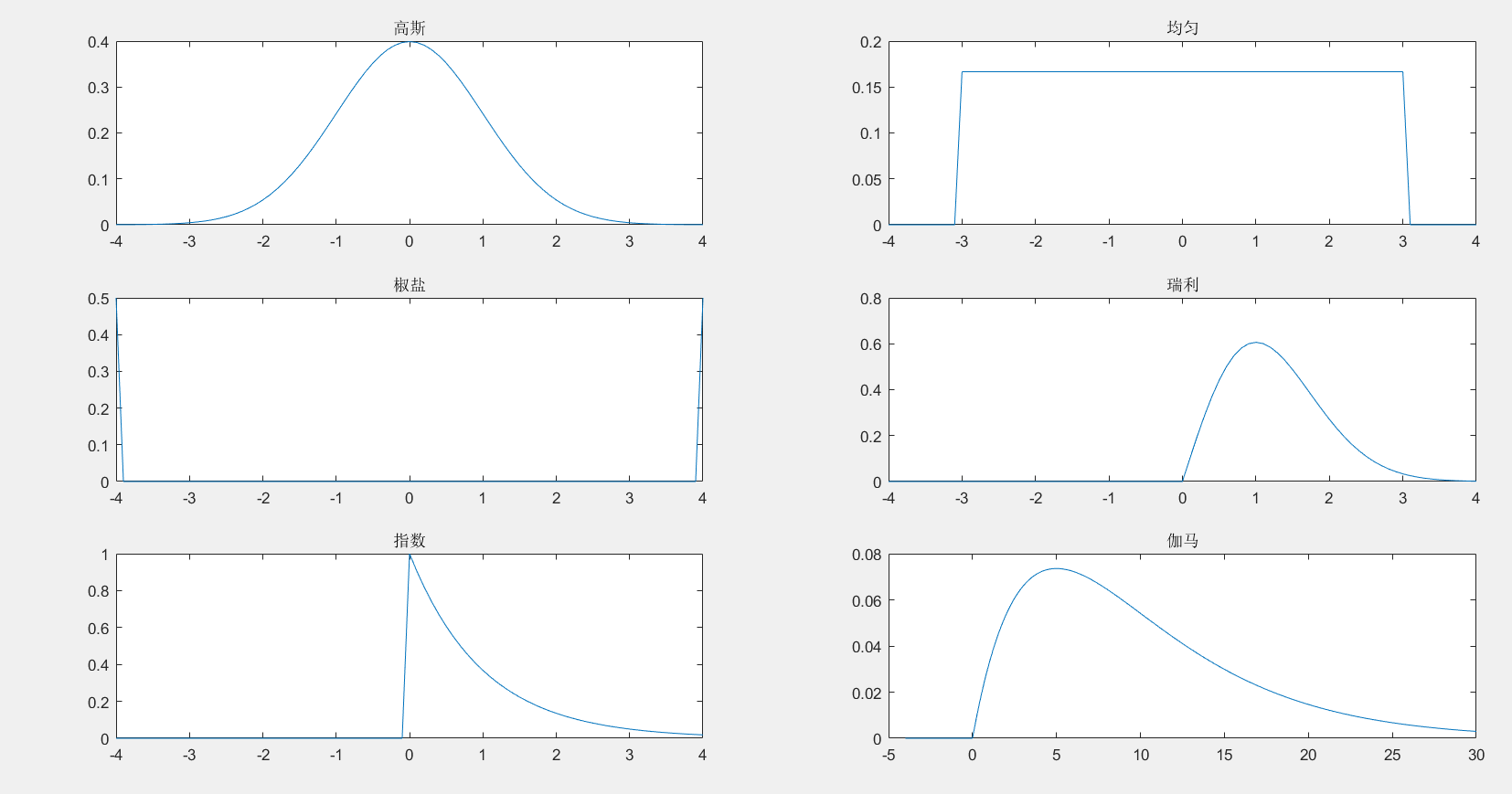## 3 为图像添加噪声

function imgOut = add_noise(imgIn,type,x,y)
% 函数功能：为图像添加噪声
% 关注微信公众号【二进制人工智能】，回复【noise】获取所有代码
% 输入：
%    imgIn：输入图形矩阵，为灰度图像
%    type:字符串，取值随噪声种类而定
%        高斯噪声：gaussian,参数为(x,y)，默认值为(0,10)
%        瑞利噪声： rayleigh,参数为x，默认值为30
%        伽马噪声： gamma,参数为(x,y),默认值为(2,10)
%        指数噪声： exp，参数为x,默认值为15
%        均匀分布： uniform，参数为(x.y)，默认值为(-20,20)
%        椒盐噪声： salt & pepper：强度为x，默认值为0.02
% 输出：
%    imgOut：添加噪声后的图像

% 预处理
if ndims(imgIn)>=3
imgIn=rgb2gray(imgIn);
end
[M,N]=size(imgIn);  % 输入图像的大小
% 设置默认噪声类型
if nargin==1
type='gaussian';
end
% 开始处理
switch lower(type)
case 'gaussian'  % 高斯噪声
if nargin<4
y=10;
end
if nargin<3
x=0;
end
% 产生高斯分布随机数
R=normrnd(x,y,M,N);
imgOut=double(imgIn)+R;
imgOut=uint8(round(imgOut));
case 'uniform' % 均匀分布
if nargin<4
y=20;
end
if nargin<3
x=-20;
end
% 产生均匀分布随机数
R=unifrnd(x,y,M,N);
imgOut=double(imgIn)+R;
imgOut=uint8(round(imgOut));
case 'salt & pepper'  % 椒盐噪声
imgOut=imnoise(imgIn,'salt & pepper',x);
if nargin<3
x=0.02;
end
a1=rand(M,N)<x;
a2=rand(M,N)<x;
imgOut=imgIn;
imgOut(a1)=0;
imgOut(a2)=255;
case 'rayleigh'        % 瑞利噪声
if nargin<3
x=30;
end
R=raylrnd(x,M,N);
imgOut=double(imgIn)+R;
imgOut=uint8(round(imgOut));
case 'exp'             % 指数噪声
if nargin<3
x=15;
end
R=exprnd(x,M,N);
imgOut=double(imgIn)+R;
imgOut=uint8(round(imgOut));
case 'gamma'   % 伽马噪声
if nargin<4
y=10;
end
if nargin<3
x=2;
end
R=gamrnd(x,y,M,N);
imgOut=double(imgIn)+R;
imgOut=uint8(round(imgOut));
otherwise
error('Unkown distribution type')
end
end


原图：

clc;clear
close all
x=uint8(zeros(256)+120);
imshow(x)### 3.1 添加高斯噪声

Y1=add_noise(x,'gaussian',0,10);
subplot(2,1,1);imshow(Y1);title('高斯')
subplot(2,1,2);hist(double(Y1(:)),200);### 3.2 添加均匀噪声

Y2=add_noise(x,'uniform',-20,20);
subplot(2,1,1);imshow(Y2);title('均匀')
subplot(2,1,2);hist(double(Y2(:)),200);### 3.3 添加椒盐噪声

Y3=add_noise(x,'salt & pepper',0.05);
subplot(2,1,1);imshow(Y3);title('椒盐')
subplot(2,1,2);hist(double(Y3(:)),200);### 3.4 添加瑞利噪声

Y4=add_noise(x,'rayleigh',30);
subplot(2,1,1);imshow(Y4);title('瑞利')
subplot(2,1,2);hist(double(Y4(:)),200);### 3.5 添加指数噪声

Y5=add_noise(x,'exp',15);
subplot(2,1,1);imshow(Y5);title('指数')
subplot(2,1,2);hist(double(Y5(:)),200);### 3.6 添加伽马噪声

figure;
subplot(2,1,1);imshow(Y6);title('伽马')
subplot(2,1,2);hist(double(Y6(:)),100);Reference

数字图像处理与机器视觉：Visual C++与Matlab实现（第二版）
作者：张铮，徐超，任淑霞，韩海玲

展开全文• 代码用于向图像添加椒盐噪声。 注意：如果你在你的系统或项目中使用我的代码，你应该总是引用我的论文作为参考 Functions: Main function : main.m Salt_and_Pepper_Noise adding function : sp.m %%%%%%%%%%%------...
• ## matlab图片添加噪音

千次阅读 2022-05-09 23:33:54
给图像噪声 type可有五种,分别为’gaussian’(高斯白噪声),’localvar’ (与图象灰度值有关的零均值高斯白噪声),’poisson’(泊松噪声), ‘salt & pepper’(椒盐噪声)和’speckle’(斑点噪声); 3、仿真分析 ...

1、内容简介

2、内容说明

给图像加噪声
type可有五种,分别为’gaussian’(高斯白噪声),’localvar’
(与图象灰度值有关的零均值高斯白噪声),’poisson’(泊松噪声),
‘salt & pepper’(椒盐噪声)和’speckle’(斑点噪声);

3、仿真分析

%%
% 给图像加噪声
% type可有五种,分别为’gaussian’(高斯白噪声),’localvar’
% (与图象灰度值有关的零均值高斯白噪声),’poisson’(泊松噪声),
% ‘salt & pepper’(椒盐噪声)和’speckle’(斑点噪声);
clear all;
close all;
clc
figure
imshow(F);title('原图');
F1 = imnoise(F,'speckle',0.003); %给图像加入噪声 0.003为可以修改的参数
figure
imshow(F1);title('加斑点噪声之后');
imwrite(F1,'speckle.jpg');
F2 = imnoise(F,'poisson'); %给图像加入噪声
figure
imshow(F2);title('加泊松噪声之后');
imwrite(F2,'poisson.jpg');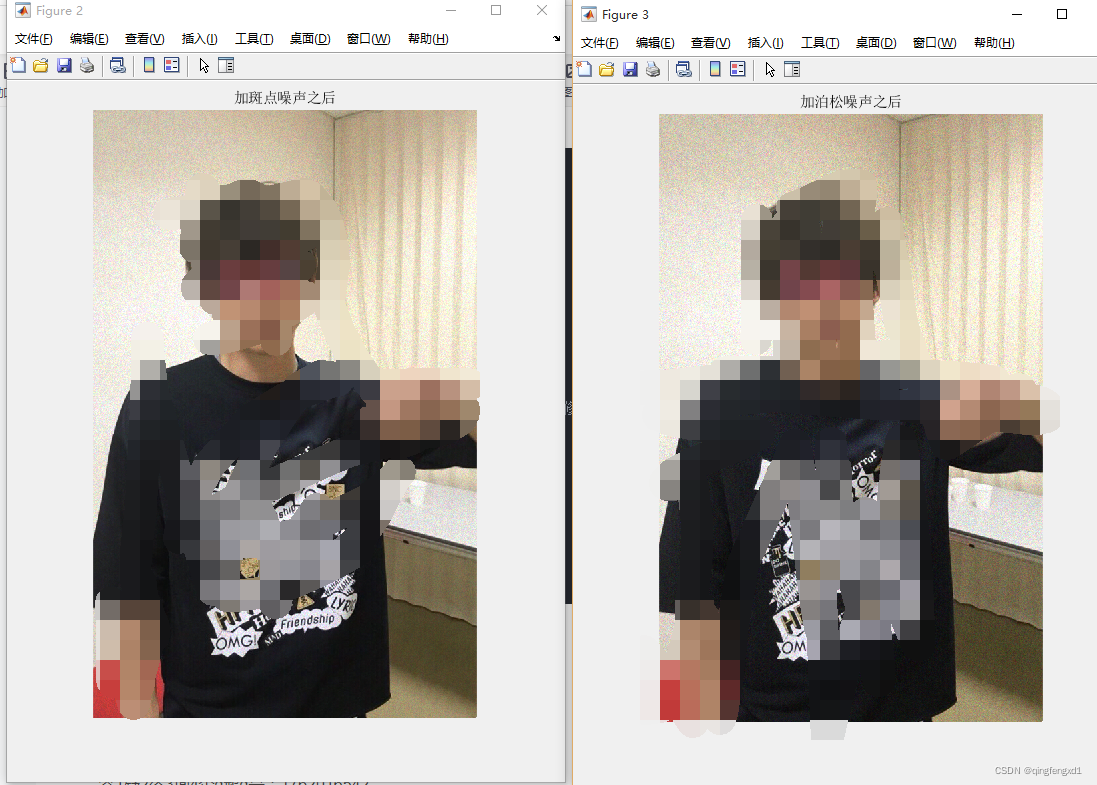4、参考论文

答1疑2咨3询4企q鹅q号：1762016542展开全文椒盐噪声
• % 在一定密度的图像添加椒盐噪声% image_noisy = 胡椒或盐 (image_orig, ND, type_noise, min_val, max_val) % image_noisy = 添加盐或胡椒或盐和胡椒噪声后的图像% image_orig = 添加盐或胡椒或盐和胡椒噪声后的...matlab
• matlab添加周期噪声代码数字图像处理-ECN316-项目 要运行Matlab代码，请按原样下载文件夹。 所有路径都是相对的，因此无需更改代码中的路径。 这是每个代码的简要说明 ●notch_filter.m-运行代码以消除noiseball.png...
• 虽然你的分少，我还是你写个完整的多功能程序供你学习：M=imread('dl011.jpg') %读取MATLAB中的名为cameraman的图像subplot(3,3,1)imshow(M) %显示原始图像title('original')P1=imnoise(M,'gaussian',0.02) %加入...
• 对tif格式图像添加椒盐噪声和高斯噪声，并显示均值滤波和中值滤波图
• ## matlab添加椒盐噪声

千次阅读 2021-10-26 20:07:42
image_matlab=imnoise(image,'salt & pepper',0.1); figure(1); subplot(131); imshow(image); title('原始图像') subplot(132); imshow(image_new); title('手写椒盐噪声') subplot(133); imsmatlab 图像处理
• ## Matlab为图像添加噪声

万次阅读 多人点赞 2019-08-28 14:31:46
img=imread('D:/pattern....添加高斯噪声 >> img_gaussian=imnoise(img, 'gaussian' , 0, 0.02 ); #均值为0，方差为0.02 >> imshow(img_gaussian); 添加泊松噪声 >> img_poisson=imnoise(i...
• 噪声分两种性质，加性噪声和乘性噪声。加性噪声一般指热噪声、散弹噪声等，它们与信号的关系是相加，不管有没有信号，噪声都存在。而乘性噪声一般由信道不理想引起，它们与信号的关系是相乘，信号在它在，信号不在他...
• 在进行数字图像去噪实验时，我们经常使用MATLAB工具去实现，本文主要讲解的是如何使用MATLAB对上述噪声进行模拟仿真。 1、高斯噪声 高斯噪声，从字面含义上可以看出，其概率密度函数服从高斯...
• matlab开发-添加噪声图像恢复。此存档包含添加噪声或恢复任何失真图像的功能。
• 在该项目中包含两个块，首先包含添加噪声块。第二件事应用该过滤器matlab
• 产生一定频率的正弦信号，并利用matlab函数加瑞利噪声和高斯噪声，模拟传输信道的衰落，最后画图对比加噪前后的信号。
• Matlab图像添加噪声和降噪报告一、课题背景 MATLABMATLAB是由美国mathworks公司发布的主要面对科学计算、可视化以及交互式程序设计的高科技计算环境。它将数值分析、矩阵计算、科学数据可视化以及非线性动态系统的...人工智能 python java 计算机视觉 机器学习
• clear all; imageA1 = imread('D:\MATLAB\bin\lena.bmp'); %读取图像 [row,col,th] = size(imageA1); %获取图像尺寸 imageA2 = double(imageA1);... %给图像加均值为0，方差为10的高斯噪声 imageA = uint8(imatlab
• MATLAB给图像添加高斯、椒盐、加性及乘性噪声[噪声生成]源代码Gaussian-Pepper-Noise-Generator
• 给图片添加了正弦噪声，利用MATLAB源代码消除噪声.rar
• Matlab图像添加噪声和降噪报告简介.docxMatlab图像添加噪声和降噪报告简介.docxMatlab图像添加噪声和降噪报告简介.docxMatlab图像添加噪声和降噪报告简介.docxMatlab图像添加噪声和降噪报告简介.docxMatlab图像添加...
• Matlab图像添加噪声和降噪报告简介.pdfMatlab图像添加噪声和降噪报告简介.pdfMatlab图像添加噪声和降噪报告简介.pdfMatlab图像添加噪声和降噪报告简介.pdfMatlab图像添加噪声和降噪报告简介.pdfMatlab图像添加噪声和...
• ## MATLAB添加噪声

万次阅读 多人点赞 2019-05-23 20:54:02
首先是用MATLAB自带的imnoise函数添加噪声。 img= im2double(imread('D:\Matlab2018a\work\img.png'));%改为你要读入图片的路径;im2double作归一化处理 sigma=25;%添加噪声的标准差 imgnoise=imnoise(img,'gaussian'...matlab 添加噪声 椒盐噪声...